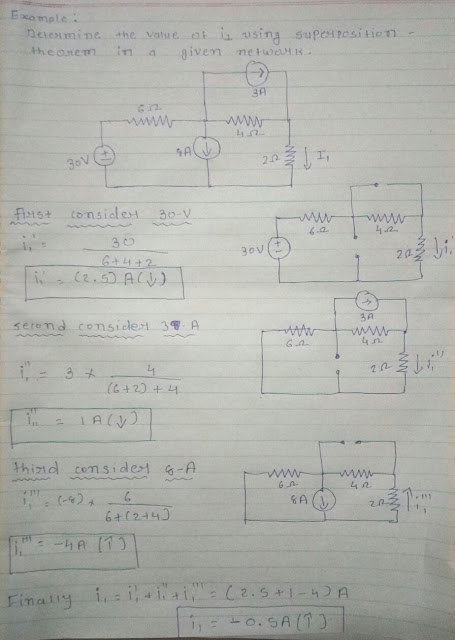# Superposition Theorem Example | Aware About Most Useful theorem For Electrical Circuit That Supper Position Theorem!!!!

## Superposition Theorem Example | Aware About Most Useful theorem For Electrical Circuit That Supper Position Theorem!!!!

### Superposition Theorem Example And How We Can Use The Supper Position Theorem In Electrical Circuit Is Given As Per Below Description

The superposition theorem or superposition priciple is

" The Superposition Theorem states that the response in any element of a linear, bialateral network containing more than one independent sources can be obtained as the algebric sum of the responses obtained by each independent source acting separately at a time and with all other independent source set equal to zero."

While evaluting the responce from one independent source other source can not be removed bodily from the circuit.

Recommended Articles :

Insted, you suppress all other Independent Source by setting their values to zero.

The suppressed source then behave like the following replacement.

1. Ideal independent voltage source - short circuit
2. Ideal independent current source - open circuit

Special care must be taken if the circuit also include the Controlled Sources.

In general a controlled source affect the individual contribution of each independent source,  Consequently Controlled Source are not suppressed during analysis by super position.
Example of Superposition Theorem calculation:Superpostion Theorem Example

Question Related Supper Position Theorem:

1) superposition theorem applicable for ________ circuit????
Ans: linear Element Circuit

2) state the superposition theorem??
Ans:  the responce in any element of a linear, bialateral network containing more than one independent sources can be obtained as the algebric sum of the responses obtained by each independent source acting separately at a time and with all other independent source set equal to zero

3)In a superposition theorem Ideal independent voltage source will be???
Ans: - short circuit

4) In superposition theorem Ideal independent current source will be????
Ans: - open circuit

5) controlled source are suppressed or not during analysis by super position????
Ans : not suppressed